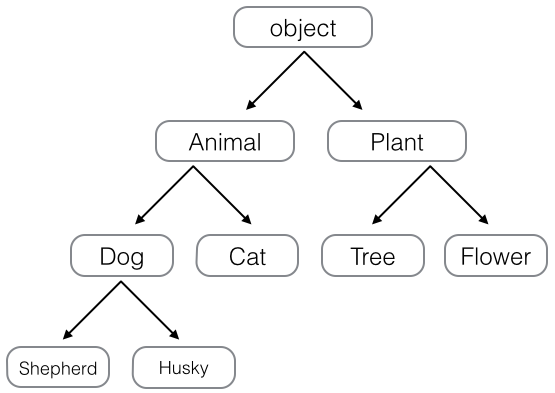# Python简介 - 廖雪峰的官方网站

#### 继承和多态

``````class Animal(object):
def run(self):
print 'Animal is running...'
``````

``````class Dog(Animal):
pass

class Cat(Animal):
pass
``````

``````dog = Dog()
dog.run()

cat = Cat()
cat.run()
``````

``````Animal is running...
Animal is running...
``````

``````class Dog(Animal):
def run(self):
print 'Dog is running...'
def eat(self):
print 'Eating meat...'
``````

``````class Dog(Animal):
def run(self):
print 'Dog is running...'

class Cat(Animal):
def run(self):
print 'Cat is running...'
``````

``````Dog is running...
Cat is running...
``````

``````a = list() # a是list类型
b = Animal() # b是Animal类型
c = Dog() # c是Dog类型
``````

``````>>> isinstance(a, list)
True
>>> isinstance(b, Animal)
True
>>> isinstance(c, Dog)
True
``````

``````>>> isinstance(c, Animal)
True
``````

``````>>> b = Animal()
>>> isinstance(b, Dog)
False
``````

Dog可以看成Animal，但Animal不可以看成Dog。

``````def run_twice(animal):
animal.run()
animal.run()
``````

``````>>> run_twice(Animal())
Animal is running...
Animal is running...
``````

``````>>> run_twice(Dog())
Dog is running...
Dog is running...
``````

``````>>> run_twice(Cat())
Cat is running...
Cat is running...
``````

``````class Tortoise(Animal):
def run(self):
print 'Tortoise is running slowly...'
``````

``````>>> run_twice(Tortoise())
Tortoise is running slowly...
Tortoise is running slowly...
``````